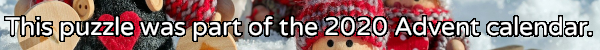mscroggs.co.uk
mscroggs.co.uksubscribe

# Puzzles

## 4 DecemberToday's number is a three digit number which is equal to the sum of the cubes of its digits. One less than today's number also has this property.

## Square and cube endings

Source: UKMT 2011 Senior Kangaroo
How many positive two-digit numbers are there whose square and cube both end in the same digit?

## What's the star?

In the Christmas tree below, the rectangle, baubles, and the star at the top each contain a number. The square baubles contain square numbers; the triangle baubles contain triangle numbers; and the cube bauble contains a cube number.
The numbers in the rectangles (and the star) are equal to the sum of the numbers below them. For example, if the following numbers are filled in:
then you can deduce the following:
What is the number in the star at the top of this tree?
You can download a printable pdf of this puzzle here.

## Archive

Show me a random puzzle
▼ show ▼
© Matthew Scroggs 2012–2021